Ray And Wave Optics MCQ Level - 1

# Ray And Wave Optics MCQ Level - 1

Test Description

## 30 Questions MCQ Test | Ray And Wave Optics MCQ Level - 1

Ray And Wave Optics MCQ Level - 1 for Class 12 2023 is part of Class 12 preparation. The Ray And Wave Optics MCQ Level - 1 questions and answers have been prepared according to the Class 12 exam syllabus.The Ray And Wave Optics MCQ Level - 1 MCQs are made for Class 12 2023 Exam. Find important definitions, questions, notes, meanings, examples, exercises, MCQs and online tests for Ray And Wave Optics MCQ Level - 1 below.
Solutions of Ray And Wave Optics MCQ Level - 1 questions in English are available as part of our course for Class 12 & Ray And Wave Optics MCQ Level - 1 solutions in Hindi for Class 12 course. Download more important topics, notes, lectures and mock test series for Class 12 Exam by signing up for free. Attempt Ray And Wave Optics MCQ Level - 1 | 30 questions in 30 minutes | Mock test for Class 12 preparation | Free important questions MCQ to study for Class 12 Exam | Download free PDF with solutions
 1 Crore+ students have signed up on EduRev. Have you?
Ray And Wave Optics MCQ Level - 1 - Question 1

### A candle is placed 20 cm from the surface of a convexmirror and a plane mirror is also placed so that thevirtual images in the two mirrors coincide. If the planemirror is 12 cm away from the object, what is the focallength of the convex mirror?

Ray And Wave Optics MCQ Level - 1 - Question 2

### A given ray of light suffers minimum deviation in anequilateral prism P. Additional prisms Q and R of identical shape and ofthe same material as P are now added as shown in Fig. The ray will suffer: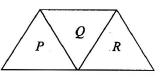Ray And Wave Optics MCQ Level - 1 - Question 3

### Consider the situation shown in Fig. Water (μ = 4/3) isfilled in a beaker upto a height of 10 cm.A plane mirror is fixed at a height of 5 cm from thesurface of water. Distance of image from the mirror afterreflection from it of an object O at the bottom of the beaker is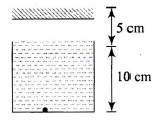Ray And Wave Optics MCQ Level - 1 - Question 4

Refraction takes place at a concave spherical boundaryseparating glass-air medium. For the image to be real,the object distance (μg = 3/2)

Ray And Wave Optics MCQ Level - 1 - Question 5

The refractive index of a prism is 2. The prism canhave a maximum refracting angle of

Ray And Wave Optics MCQ Level - 1 - Question 6

Angle of minimum deviation is equal to the angle ofprism of an equilateral glass prism. The angle ofincidence at which minimum deviation will be obtained is

Ray And Wave Optics MCQ Level - 1 - Question 7

A plano-convex lens fits exactly into a plano-concavelens. Their plane surfaces are parallel to each other. Ifthe lenses are made of different material of refractiveindices μ1 and μand R is the radius of curvature of the curved surface of the lenses, then focal length of thecombination is

Ray And Wave Optics MCQ Level - 1 - Question 8

A parallel beam of light is incident on the system oftwo convex lenses of focal lengths f1 = 20 cm and f2 = 10 cm. What should be the distance between thetwo lenses so that rays after refraction from both thelenses pass undeviated?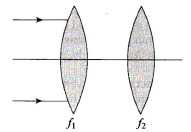Ray And Wave Optics MCQ Level - 1 - Question 9

A plano-convex glass lens (μg = 3/2) of radius ofcurvature R = 10 cm is placed at a distance of ‘b’ froma concave lens of focal length 20 cm.What should be the distance ‘a’ of a point object Ofrom the plano-convex lens so that the position of finalimage is independent of’b’?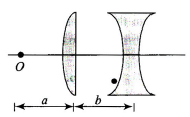Ray And Wave Optics MCQ Level - 1 - Question 10

A point object is placed at a distance of 25 cm from aconvex lens of focal length 20 cm. If a glass slab ofthickness t and refractive index 1.5 is inserted between the lens and the object, the image is formed at infinity. The thickness t is

Ray And Wave Optics MCQ Level - 1 - Question 11

A convex lens of focal length 10 cm is painted black atthe middle portion as shown in Fig. An object is placedat a distance of 20 cm from the lens.Then,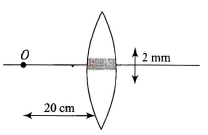Ray And Wave Optics MCQ Level - 1 - Question 12

Consider an equiconvex lens of radius of curvature Rand focal length f. If f > R, the refractive index μ of thematerial of the lens

Ray And Wave Optics MCQ Level - 1 - Question 13

An equiconvex lens is cut into two halves along (i) XOX’ and (ii) YOY” as shown in Fig. Let f, f ' ,f '' be the focal length of the lens, of each half in case (i), and of each half in case (ii), respectively, Choose the correct statement from the following: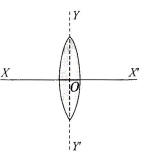Ray And Wave Optics MCQ Level - 1 - Question 14

A spherical mirror forms an image of magnification 3.The object distance, if focal length of mirror is 24 cm,may be

Ray And Wave Optics MCQ Level - 1 - Question 15

The critical angle for light going from medium X intomedium Y is θ . The speed of light in medium X is v.The speed of light in medium Y is

Ray And Wave Optics MCQ Level - 1 - Question 16

A convex lens of focal length 1.0 m and a concave lens of focal length 0.25 m are 0.75 m apart. A parallel beam of light is incident on the convex lens. The beam emerging after refraction from both lenses is

Ray And Wave Optics MCQ Level - 1 - Question 17

A convex lens A of focal length 20 cm and a concavelens G of focal length 5 cm are kept along the sameaxis with the distance d between them. If a parallelbeam of light falling on A leaves B as a parallel beam,then distance d in cm will be

Ray And Wave Optics MCQ Level - 1 - Question 18

A convex lens forms an image of an object placed 20cm away from it at a distance of 20 cm on the otherside of the lens. If the object is moves 5 cm toward thelens, the image will be

Ray And Wave Optics MCQ Level - 1 - Question 19

With a concave mirror, an object is placed at a distance x1 from the principal focus, on the principal axis. The image is formed at a distance x2 from the principal focus. The focal length of the mirror is

Ray And Wave Optics MCQ Level - 1 - Question 20

A concave mirror is placed on a horizontal table withits axis directed vertically upward. Let O be the pole ofthe mirror and C its center of curvature. A point objectis placed at C. It has a real image, also located at C. Ifthe mirror is now filled with water, the image will be

Ray And Wave Optics MCQ Level - 1 - Question 21

A point source of light B is placed at a distance L infront of the center of a mirror of width d hung verticallyon a wall. A man walks in front of the mirror along a lineparallel to the mirror at a distance 2L from it as shownin Fig.the greatest distance over which he can see theimage of the light source in the mirror is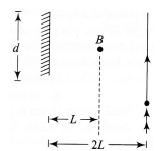Ray And Wave Optics MCQ Level - 1 - Question 22

When an object is kept at a distance of 30 cm from aconcave mirror, the image is formed at a distance of10 cm. If the object is moved with a speed of 9 cm s-1 the speed with which the image moves is

Ray And Wave Optics MCQ Level - 1 - Question 23

A convex mirror of radius of curvature 1.6 m has an object placed at a distance of 1 m from it. The image is formed at a distance of

Ray And Wave Optics MCQ Level - 1 - Question 24

In the above question, the magnification is

Ray And Wave Optics MCQ Level - 1 - Question 25

A convex mirror and a concave mirror of radius 10 cmeach are placed 15 cm apart facing each other. Anobject is placed midway between them. If the reflectionfirst takes place in the concave mirror and then inconvex mirror, the position of the final image is

Ray And Wave Optics MCQ Level - 1 - Question 26

A small piece of wire bent into an L shape, with uprightand horizontal portions of equal lengths, is placed withthe horizontal portion along the axis of the concavemirror whose radius of curvature is 10 cm. If the bendis 20 cm from the pole of the mirror, then the ratio ofthe lengths of the images of the upright and horizontalportions of the wire is

Ray And Wave Optics MCQ Level - 1 - Question 27

The image of an object placed on the principal axis ofa concave mirror of focal length 12 cm is formed at apoint which is 10 cm more distance from the mirror than the object. The magnification of the image is

Ray And Wave Optics MCQ Level - 1 - Question 28

A clear transparent glass sphere (μ =1.5) of radius R is immersed in a liquid of refractive index 1.25. A parallel beam of light incident on it will converge to a point. The distance of this point from the center will be

Ray And Wave Optics MCQ Level - 1 - Question 29

A ray of light traveling in glass (μ = 3/2) is incident ona horizontal glass-air surface at the critical angle θC Ifa thin layer of water (μ = 4/3) is now poured on theglass-air surface, the angle at which the ray emergesinto air at the water-air surface is

Ray And Wave Optics MCQ Level - 1 - Question 30

A ray of light enters a rectangular glass slab of refractiveindex√3 at an angle of incidence 60°. It travels adistance of 5 cm inside the slab and’ emerges out ofthe slab. The perpendicular distance between theincident and the emergent rays is

 Use Code STAYHOME200 and get INR 200 additional OFF Use Coupon Code
Information about Ray And Wave Optics MCQ Level - 1 Page
In this test you can find the Exam questions for Ray And Wave Optics MCQ Level - 1 solved & explained in the simplest way possible. Besides giving Questions and answers for Ray And Wave Optics MCQ Level - 1, EduRev gives you an ample number of Online tests for practice